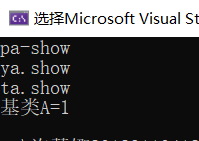# 多态、虚函数

1.（1）派生类和基类的成员函数同名的处理

#include<iostream>
#include<string>
using namespace std;
class A {
private:
int a;
public:
A(int x) { a = x; }
void setA(int x) { a = x; }
void show() { cout << "基类A=" << a << endl; }
};
class C :public A {  //公有继承 A
private:
int c;
public:
C(int a, int b, int c1) :A(a) { c = c1; }  //派生类构造函数
void setC(int x, int y) { c = x; }
void showC() { cout << "c=" << c << endl; }
/*void show() { cout << "派生类C=" << c << endl; }*/
void show() {
A::show();
cout << "派生类C=" << c << endl;
}

};
void main() {
A  ta(12);
C  tc(1, 2, 3);
ta.show();
tc.show();
}

▲main函数中的ta.show() 和tc.show()分别调用的哪个类中的show函数？

▲将C类中的show函数修改为：

void show()  {

A::show();

cout<<"派生类C="<<c<<endl;

}①子类中存在和基类中同名同参的函数，这时候基类的函数会被子类的函数覆盖，直接用子类对象调用同名函数会默认调用子类的函数。

②当子类中的函数与基类的函数，函数名相同，形参的个数或类型不同时，不能直接用子类对象调用（直接调用编译也会提示错误）。

此时要从子类中访问基类的函数有两种方法：

1、定义基类指针，让基类指针指向派生类对象，用指针调用基类函数。

2、显示调用基类函数，既在基类函数之前加上基类名和域说明符。

（2）赋值兼容原则

#include<iostream>
#include<string>
using namespace std;
class A  {
private:
int a;
public:
A(int x)  {   a=x;   }
void setA(int x){ a = x; }
void show(){  cout<<"基类A="<<a<<endl;  }
};
class C:public A   {  //公有继承A
private:
int c;
public:
C(int a,int c1):A(a)  {   c=c1;   }  //派生类构造函数
void setC(int x)  {  c = x;  }
void showC()  {  cout<<"c="<<c<<endl;  }
void show()  {  //A::show();  cout<<"派生类C="<<c<<endl;  }
};
void main()  {
A  ta(12),*pa;
C  tc(1,3);
A  &ya=tc;  //赋值兼容原则
pa=&tc;
cout<<"pa-show"<<endl;
pa->show();  //输出什么结果
cout<<"ya.show"<<endl;
ya.show(); //输出什么结果
ta=tc;
cout<<"ta.show"<<endl;
ta.show(); //输出什么结果
}

2） 预测输出结果，然后运行，说明main函数中的三个show函数分别调用的是哪个类中的show成员函数。

3）将类A中的show函数改为虚函数：virtual void show()  {  cout<<"基类A="<<a<<endl;}

2、ta=tc#include<iostream>
using namespace std;
const float PI = 3.1416;
class base {
public:
virtual void area()
{
cout << "base::area()" << endl;
}
};
class Triangle :public base {
public:
Triangle() {
cout << "请输入三角形的底边a和高h:" << endl;
cin >> a >> h;
}
void area() {
cout << "三角形的面积是：" << a * h / 2 << endl;
}
private:
float a, h;
};
class Circle:public base {
public:
Circle() {
cout << "请输入圆的半径：" << endl;
cin >> r;
}
void area() {
cout << "圆的面积是：" << PI * r * r << endl;
}
private:
float r;
};
int main() {
base* b1, * b2;//定义一个指向基类对象的指针变量，并使它指向同一类族中需要调用该函数的对象。
b1 = new Triangle;
b2 = new Circle;
b1->area();
b2->area();
return 0;
}

posted @ 2020-02-13 22:34  nanaa  阅读(151)  评论(0编辑  收藏  举报